# Olympiad Test: Analytical Reasoning - 1

## 10 Questions MCQ Test Mathematical Olympiad Class 8 | Olympiad Test: Analytical Reasoning - 1

Description
Attempt Olympiad Test: Analytical Reasoning - 1 | 10 questions in 20 minutes | Mock test for Class 8 preparation | Free important questions MCQ to study Mathematical Olympiad Class 8 for Class 8 Exam | Download free PDF with solutions
QUESTION: 1

### Direction: In each of the following problems, find the number of triangles in the given figure: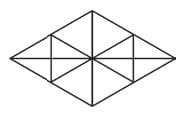Solution:

12 + 8 + 4 + 4 = 28

QUESTION: 2

### Direction: In each of the following problems, find the number of triangles in the given figure: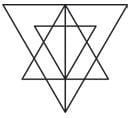Solution:

8 + 10 + 5 + 2 + 1 + 1 = 27.

QUESTION: 3

### Direction: In each of the following problems, find the number of triangles in the given figure: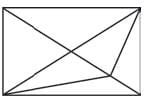Solution:

6 + 6 + 2 + 1 + 15

QUESTION: 4

Direction: In each of the following problems, find the number of triangles in the given figure: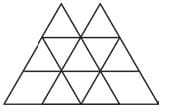Solution:

10 + 2 + 4 + 2 = 18

QUESTION: 5

Direction: In each of the following problems, find the number of triangles in the given figure: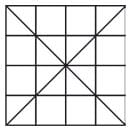Solution:

16 + 4 + 8 + 4 + 4 + 8 + 4 = 48

QUESTION: 6

Direction: In each of the following problems, find the number of triangles in the given figure: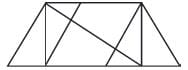Solution:

6 + 4 + 3 + 1 = 14

QUESTION: 7

Direction: In each of the following problems, find the number of triangles in the given figure: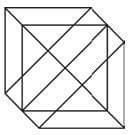Solution:
QUESTION: 8

Direction: In each of the following problems, find the number of triangles in the given figure: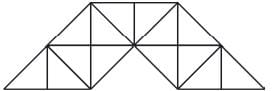Solution:
QUESTION: 9

Direction: In each of the following problems, find the number of triangles in the given figure: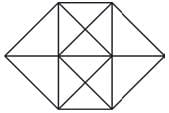Solution:
QUESTION: 10

Direction: In each of the following problems, find the number of triangles in the given figure: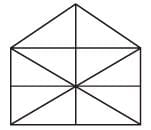Solution:Use Code STAYHOME200 and get INR 200 additional OFF Use Coupon Code